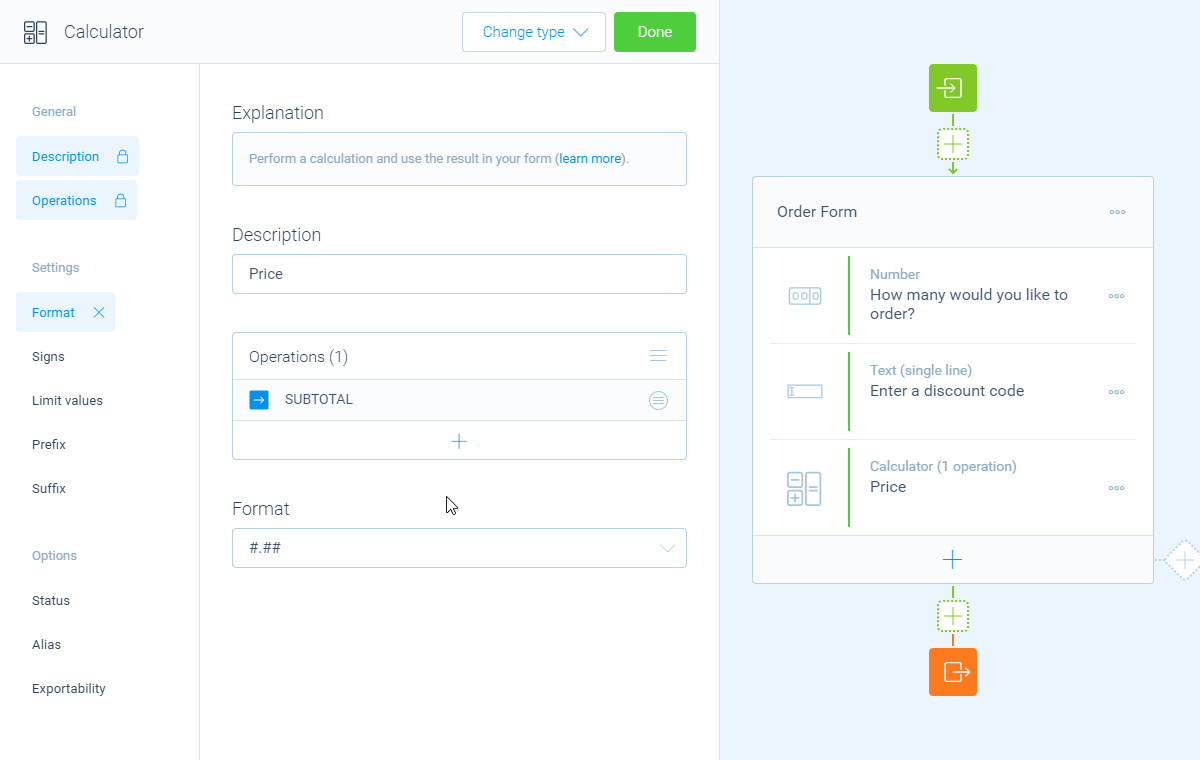Using logic5 Min.

# How to use comparisons in your calculations

You can use comparisons inside each calculation block to perform operations based on the outcome of the comparison, for example to check a discount code.

## When to use

Use the calculator block when you want to perform calculations inside your form. You can use comparisons to check certain values and based on the outcome of the comparison, perform a certain operator in your calculation. For example:

• Compare an entered discount code and perform the discount if the discount code is correct;
• Compare the total price of a cart and add up the shipment costs if the price is below a certain amount.

These are just some examples. Basically you can calculate anything you want with the calculator block. Please have a look at our calculator features overview to see everything you can do with the calculator block.

## How to use

In the article you're reading now we describe how to use comparisons in calculator blocks. For global instructions about the calculator block, please have a look at this article.

You can use comparisons inside each operation. From the menu to add an operation, you will see a menu item `Comparison` (if you have an empty form, you will see these menus directly). This lists all blocks in your form that support comparisons. From that list, select your question block that you want to compare.

### Setup comparison

You can compare basically everything with each other and use those comparisons to calculate the right values. Each comparison contains of a few steps, namely:

1. Input;
2. Compare mode;
3. Compare filter;
4. Outcome.

#### Input

First step is to determine what has to be compared. The following options are available to use as input for comparison:

• `Last outcome (ANS)` - Compare the last outcome of the calculator with another value;
• `Number` - Compare a number with another value;
• `Date/time` - Compare the current date (and time) with another moment;
• `Value (given answer)` - Compare a given answer of your respondents to a certain question with another value.

#### Compare mode

Second step is to determine how you want to compare. It depends on what you are comparing, but there are always several compare modes. For example if you're comparing with numbers:

• `Equal to ...`;
• `Lower than ...`;
• `Higher than ...`;
• `Between ... and ...`.

Specific compare modes are built in for other types of comparisons, for example for text comparisons and date comparisons.

#### Compare filter

Third step is to determine to what you want to compare to. You can compare the selected input with all different kinds of values:

• `Last outcome (ANS)`;
• `Number`Enter a static number;
• `Value (given answer)`Select a question from your form;
• `Constant`Select a built-in constant value.

#### Outcome

Last step, you determine the desired outcomes of the comparison. There are two possible outcomes:

• A value when the comparison is true;
• A value when the comparison is false.

And for each outcome, you again can use all different kinds of values:

• `Last outcome (ANS)`;
• `Number`Enter a static number;
• `Value (given answer)`Select a question from your form;
• `Constant`Select a built-in constant value.

Those outcomes will be used as the value for the operation.In this example we compare a supplied text with value DISCOUNT. If the comparison is true, a discount of \$4.99 is subtracted. Else no discount is subtracted.

Please have a look at our calculator features overview to see all comparisons you can use in the calculator block.

## Example: subtract a discount

A good example to use a comparison is to check if your customer has entered a valid coupon code, in this case coupon code `DISCOUNT`. And if that code is entered subtract a discount to the shopping cart. Let's see how we can set this up.

#### 1. Create shopping form

First we have created our shopping form. It's a pretty easy form where you can enter the number of products you'd like to order. At the end there is a text input field where customers can enter a discount code.

Now, at the end of the form we want to calculate the total price and optionally subtract a discount. To do so, we add a `Calculator` block and we give that block a name, for example `Price`.

For this example we have already setup the pricing calculations, so we move on to the comparison.

#### 3. Compare discount code

We now want to compare the supplied discount code. If the discount code matches a valid code, then we subtract a discount of € 4.99. To do that, we create a `Subtract` operation with type `Comparison`Select `Blocks`Select our discount input question.

Now we setup the comparison: if the entered text `matches 'DISCOUNT'`, then `output a fixed number of 4.99`, else `output a fixed number of 0`.

Now that we have subtracted the possible discount, the calculator block contains the total price.

#### 4. Show total price

Now we want to show the total price to our respondents in the closing message. To do so, we open up the closing message. By typing the ` @ ` sign you can select our calculator block that calculates the total price. Now the price will be shown in that position.

The calculator block has lots of features, so we have several ways to learn all about it.

### Help center

Our help articles help you out on all different aspects of the calculator:

### Overviews

We also made some overviews of the capabilities that the calculator block provides: